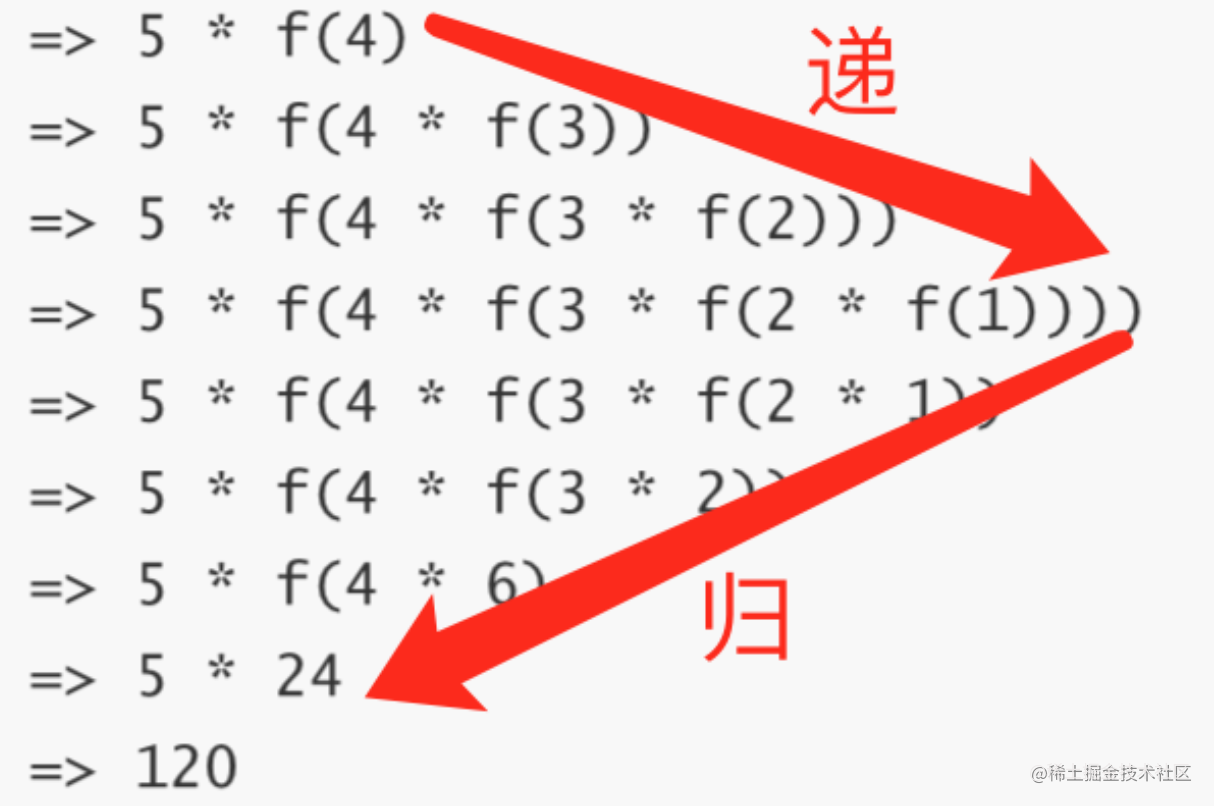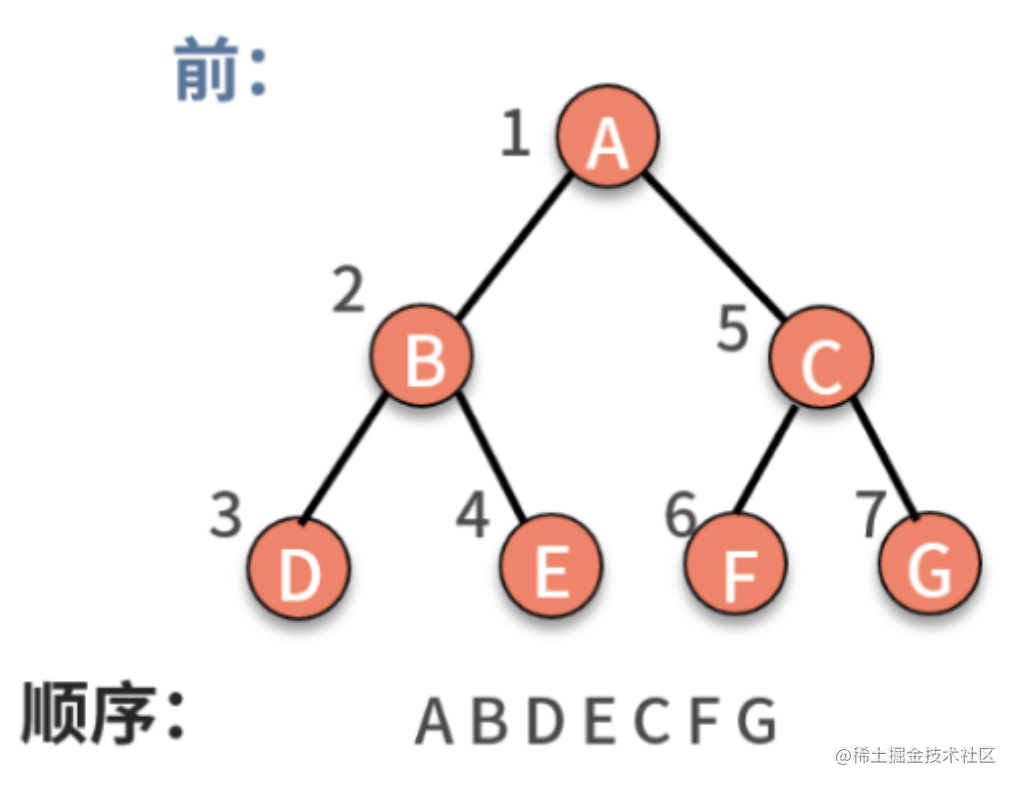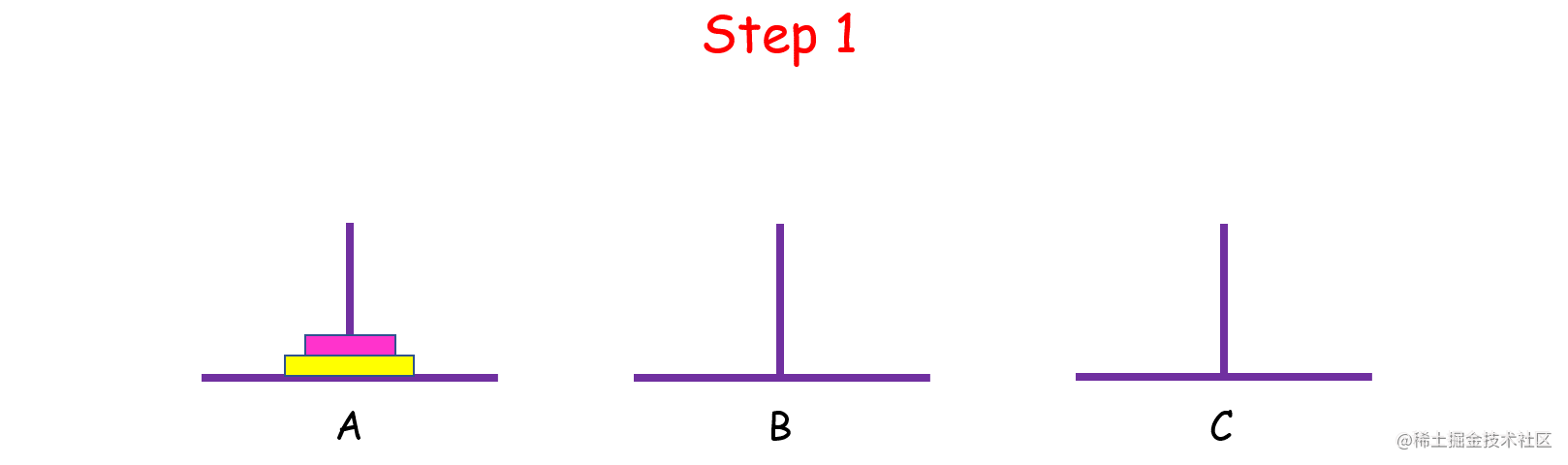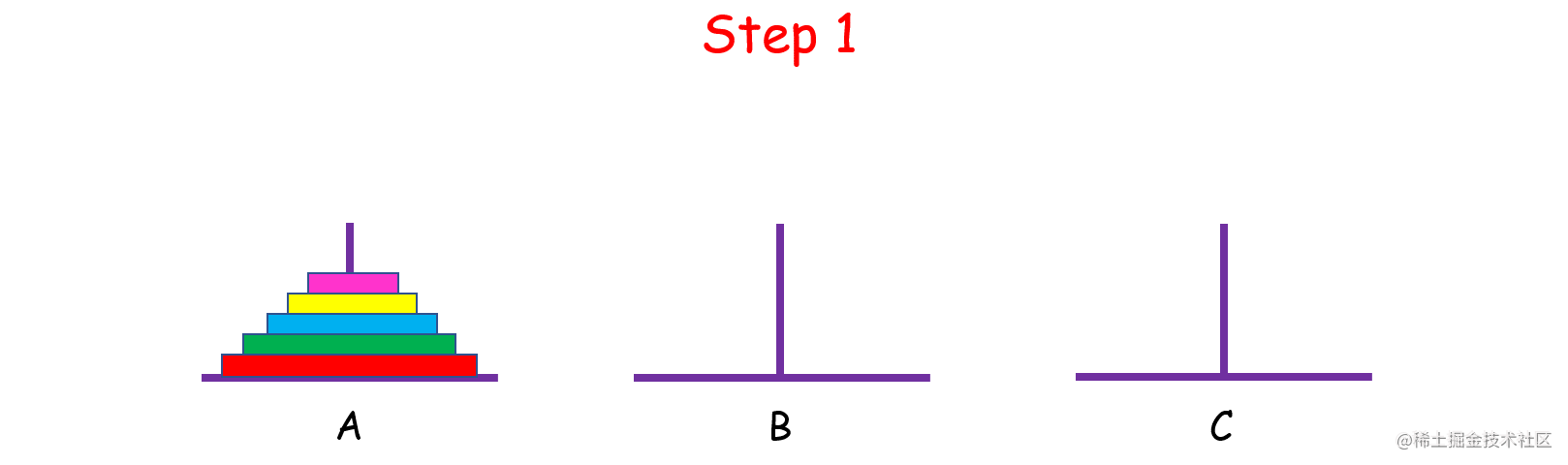# 理解递归

## 递归的概述

• 举个语言例子：

``````func1() {
大雄在房里，用时光电视看着从前的情况。电视画面中的那个时候，他正在房里，用时光电视，看着  从前的情况。电视画面中的电视画面的那个时候，他正在房里，用时光电视，看着从前的情况……
func1()
}

## 程序员眼中的递归

• 递归问题必须可以分解为若干个规模较小、与原问题形式相同的子问题。并且这些子问题可以用完全相同的解题思路来解决；
• 递归问题的演化过程是一个对原问题从大到小进行拆解的过程，并且会有一个明确的终点（临界点）。一旦原问题到达了这个临界点，就不用再往更小的问题上拆解了。最后，从这个临界点开始，把小问题的答案按照原路返回，原问题便得以解决。

``````func factorial(_ n: Int)->Int {
if 1 == n {
return n;
} else {
return factorial(n-1) * n
}
}

``````factorial简化为f

factorial(5)
=> 5 * f(4)
=> 5 * f(4 * f(3))
=> 5 * f(4 * f(3 * f(2)))
=> 5 * f(4 * f(3 * f(2 * f(1))))
=> 5 * f(4 * f(3 * f(2 * 1))
=> 5 * f(4 * f(3 * 2))
=> 5 * f(4 * 6)
=> 5 * 24
=> 120看图理解递归就是，先一步步往下递，然后回归，回归的起点就是达到终止条件的时候。

## 递归的算法思想

1. 证明当 n = 1 时命题成立；
2. 假设 n = m 时命题成立，那么尝试推导出在 n = m + 1 时命题也成立。

1. 当你面对一个大规模问题时，如何把它分解为几个小规模的同样的问题；
2. 当你把问题通过多轮分解后，最终的结果，也就是终止条件如何定义。

1. 可以拆解为除了数据规模以外，求解思路完全相同的子问题；
2. 存在终止条件。

## 递归的案例

1，前序遍历二叉树，如下图所示：• 对树中的任意结点来说，先打印这个结点，然后前序遍历它的左子树，最后前序遍历它的右子树。

``````func preOrderTraverse(_ root: TreeNode?) {
//终止条件
guard let rt = root else {
return
}

//遍历步骤
print("node:(rt.val)")
preOrderTraverse(rt.left)
preOrderTraverse(rt.right)
}

2，汉诺塔问题是源于印度一个古老传说的益智玩具。大梵天创造世界的时候做了三根金刚石柱子，在一根柱子上从下往上按照大小顺序摞着 64 片黄金圆盘。大梵天命令婆罗门把圆盘从下面开始按大小顺序重新摆放在另一根柱子上，并且规定，在小圆盘上不能放大圆盘，在三根柱子之间一次只能移动一个圆盘。

• 假设 n = 1,只有一个盘子，很简单，直接把它从 A 中拿出来，移到 C 上；
• 如果 n = 2 呢？这时候我们就要借助 B 了，因为小盘子必须时刻都在大盘子上面，共需要 4 步。• n = 1 时，直接把盘子从 A 移到 C；

• n > 1 时，

• 先把上面 n - 1 个盘子从 A 移到 B（子问题，递归）；
• 再将最大的盘子从 A 移到 C；
• 再将 B 上 n - 1 个盘子从 B 移到 C（子问题，递归）。

``````class Solution {
func hanota(_ A: inout [Int], _ B: inout [Int], _ C: inout [Int]) {

let n = A.count
move(n, &A, &B, &C)

}

func move(_ n:Int,_ A: inout [Int], _ B: inout [Int], _ C: inout [Int])       {
if n == 1{
C.append(A[A.count-1])
A.removeLast()
return
}
else{
move(n-1,&A, &C, &B)

C.append(A[A.count-1])
A.removeLast()

move(n-1,&B, &A, &C)
}
}

}